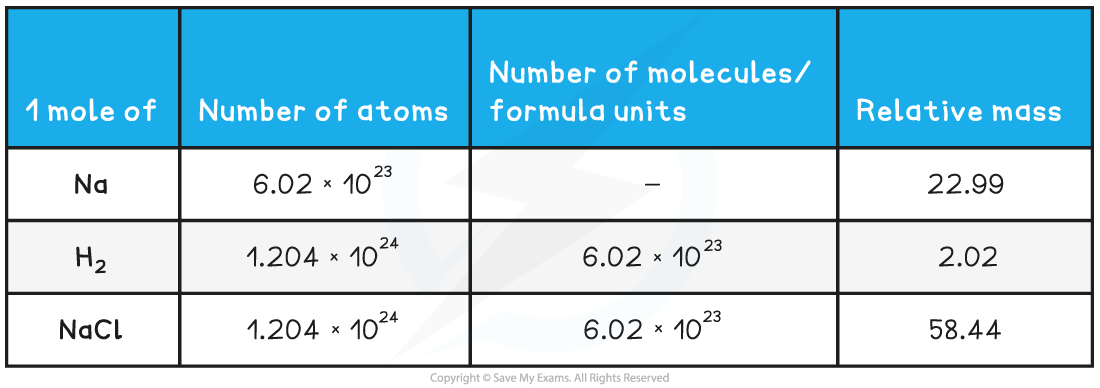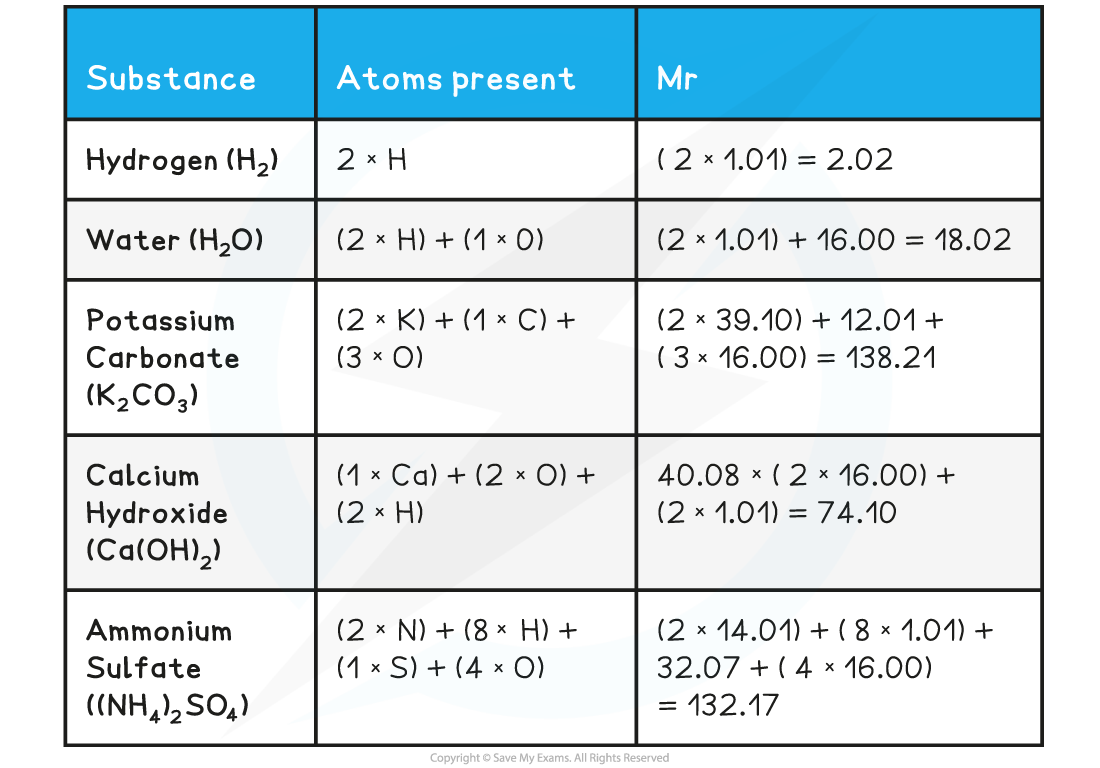# IB DP Chemistry: SL复习笔记1.1.4 The Mole Concept

### The Mole

• The Avogadro constant (NA or L) is the number of particles equivalent to the relative atomic mass or molecular mass of a substance in grams
• The Avogadro constant applies to atoms, molecules and ions
• The value of the Avogadro constant is 6.02 x 1023 g mol-1
• The mass of a substance with this number of particles is called the molar mass
• One mole of a substance contains the same number of fundamental units as there are atoms in exactly 12.00 g of 12C
• If you had 6.02 x 1023 atoms of carbon-12 in your hand, you would have a mass of exactly 12.00 g
• One mole of water would have a mass of (2 x 1.01 + 16.00) = 18.02 g

#### Worked Example

Determine the number of atoms, molecules and the relative mass of 1 mole of:

1. Na
2. H2
3. NaCl

• The relative atomic mass of Na is 22.99
• Therefore, 1 mol of Na has a mass of 22.99 g mol-1
• 1 mol of Na will contain 6.02 x 1023 atoms of Na (Avogadro’s constant)

• The relative atomic mass of H is 1.01
• Since there are 2 H atoms in H2, the mass of 1 mol of H2 is (2 x 1.01) 2.02 g mol-1
• 1 mol of H2 will contain 6.02 x 1023 molecules of H2
• However, since there are 2 H atoms in each molecule of H2, 1 mol of H2 molecules will contain 1.204 x 1024 H atoms

• The relative atomic masses of Na and Cl are 22.99 and 35.45 respectively
• Therefore, 1 mol of NaCl has a mass of (22.99 + 35.45) 58.44 g mol-1
• 1 mol of NaCl will contain 6.02 x 1023  formula units of NaCl
• Since there is both an Na and a Cl atom in NaCl, 1 mol of NaCl will contain 1.204 x 1024 atoms in total### Relative Mass

#### Relative atomic mass, Ar

• The relative atomic mass (Ar) of an element is the weighted average mass of one atom compared to one twelfth the mass of a carbon-12 atom
• The relative atomic mass is determined by using the weighted average mass of the isotopes of a particular element
• The Ar has no units as it is a ratio and the units cancel each other out#### Relative isotopic mass

• The relative isotopic mass is the mass of a particular atom of an isotope compared to one twelfth the mass of a carbon-12 atom
• Atoms of the same element with a different number of neutrons are called isotopes
• Isotopes are represented by writing the mass number as 20Ne, or neon-20 or Ne-20
• To calculate the average atomic mass of an element the percentage abundance is taken into account
• Multiply the atomic mass by the percentage abundance for each isotope and add them all together
• Divide by 100 to get average relative atomic mass
• This is known as the weighted average of the masses of the isotopes#### Relative molecular mass, Mr

• The relative molecular mass (Mr) is the weighted average mass of a molecule compared to one twelfth the mass of a carbon-12 atom
• The Mr has no units• The Mcan be found by adding up the relative atomic masses of all atoms present in one molecule
• When calculating the Mr the simplest formula for the compound is used, also known as the formula unit
• E.g. Silicon dioxide has a giant covalent structure, but the simplest formula (the formula unit) is SiO2#### Relative formula mass, Mr

• The relative formula mass (Mr) is used for compounds containing ions
• It has the same units and is calculated in the same way as the relative molecular mass
• In the table above, the Mfor potassium carbonate, calcium hydroxide and ammonium sulfate are relative formula masses